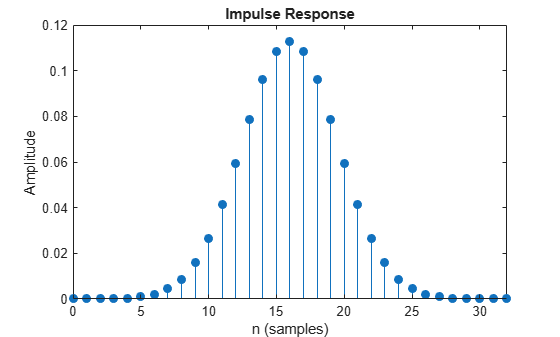# gaussdesign

Gaussian FIR pulse-shaping filter design

## Syntax

``h = gaussdesign(bt,span,sps)``

## Description

example

````h = gaussdesign(bt,span,sps)` designs a lowpass FIR Gaussian pulse-shaping filter and returns a vector `h` of filter coefficients. The filter is truncated to `span` symbols, and each symbol period contains `sps` samples. The order of the filter, `sps*span`, must be even.```

## Examples

collapse all

Specify that the modulation used to transmit the bits is a Gaussian minimum-shift keying (GMSK) pulse. This pulse has a 3-dB bandwidth equal to 0.3 of the bit rate. Truncate the filter to 4 symbols and represent each symbol with 8 samples.

```bt = 0.3; span = 4; sps = 8; h = gaussdesign(bt,span,sps); fvtool(h,'impulse')```## Input Arguments

collapse all

Product of the 3-dB one-sided bandwidth and the symbol time, specified as a positive real scalar. The 3-dB one-sided bandwidth is in hertz and the symbol time is in seconds. Smaller values of `bt` produce larger pulse widths.

Number of symbols, specified as a positive integer scalar.

Number of samples per symbol period (oversampling factor), specified as a positive integer scalar.

## Output Arguments

collapse all

FIR coefficients of the Gaussian pulse-shaping filter, returned as a row vector. The coefficients are normalized so that the nominal passband gain is always 1.

Data Types: `double`

## Algorithms

The impulse response of the Gaussian filter is given by

`$h\left(t\right)=\frac{\mathrm{exp}\left(\frac{-{t}^{2}}{2{\delta }^{2}}\right)}{\sqrt{2\pi }\cdot \delta }$`

where

`$\delta =\frac{\sqrt{\mathrm{log}2}}{2\pi BT}.$`

BT is the bandwidth-symbol time product specified in `bt`, where B is the 3-dB bandwidth of the filter and T is the symbol time. The number of symbols between the start and end of the impulse (`span`) and the number of samples per symbol (`sps`) determine the length of the impulse response: $span×sps+1.$